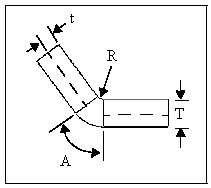# K-Factor

K-Factor is a ratio that represents the location of the neutral sheet with respect to the thickness of the sheet metal part.

When you select K-Factor as the bend allowance, you can specify a K-Factor bend table. The SOLIDWORKS application also comes with a K-Factor bend table in Microsoft Excel format. This is located in install_dir\lang\English\Sheetmetal Bend Tables\kfactor base bend table.xls.

You can specify different values for the K-Factor in different configurations.
You can also apply a material-based default K-Factor by using a Sheet Metal Gauge table.

Bend allowance with a K-Factor is calculated as follows:

BA=pi(R + KT) A/180

where:

 BA = bend allowance R = inside bend radius K = K-Factor, which is t / T T = material thickness t = distance from inside face to neutral sheet A = bend angle in degrees (the angle through which the material is bent)